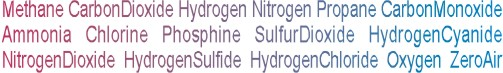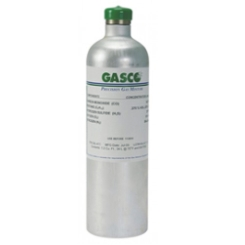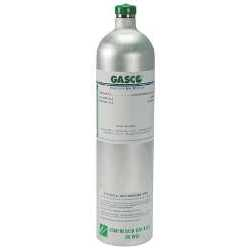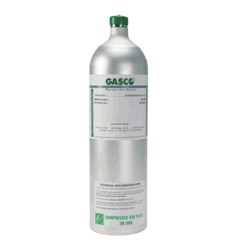Calibration gas blends, mixes and accessories
REACTIVE SINGLE GAS > NITROGEN DIOXIDE :    17 Items found.(1490)

NORLAB Equivalent  F106710

Norlab Equivalent Calibration Gas 10 ppm Nitrogen Dioxide Balance nitrogen 34L Liter cylinder (Made by Gasco)

Configure

\$199.77(1491)

NORLAB Equivalent  F106725A

Norlab Equivalent Calibration Gas 25 ppm Nitrogen Dioxide Balance air 34L Liter cylinder (Made by Gasco)

Configure

\$199.77(1492)

NORLAB Equivalent  F106725

Norlab Equivalent Calibration Gas 25 ppm Nitrogen Dioxide Balance nitrogen 34L Liter cylinder (Made by Gasco)

Configure

\$199.77(1493)

NORLAB Equivalent  F106750A

Norlab Equivalent Calibration Gas 50 ppm Nitrogen Dioxide Balance air 34L Liter cylinder (Made by Gasco)

Configure

\$199.77(1489)

NORLAB Equivalent  F106710A

Norlab Equivalent Calibration Gas 10 ppm Nitrogen Dioxide Balance air 34L Liter cylinder (Made by Gasco)

Configure

\$199.77(1498)

NORLAB Equivalent  Z106725A

Norlab Equivalent Calibration Gas 25 ppm Nitrogen Dioxide Balance air 58L Liter cylinder (Made by Gasco)

Configure

\$281.29(1494)

NORLAB Equivalent  Z10675A

Norlab Equivalent Calibration Gas 5 ppm Nitrogen Dioxide Balance air 58L Liter cylinder (Made by Gasco)

Configure

\$281.29(1495)

NORLAB Equivalent  Z10675

Norlab Equivalent Calibration Gas 5 ppm Nitrogen Dioxide Balance nitrogen 58L Liter cylinder (Made by Gasco)

Configure

\$281.29(1497)

NORLAB Equivalent  Z106710

Norlab Equivalent Calibration Gas 10 ppm Nitrogen Dioxide Balance nitrogen 58L Liter cylinder (Made by Gasco)

Configure

\$281.29(1499)

NORLAB Equivalent  Z106725

Norlab Equivalent Calibration Gas 25 ppm Nitrogen Dioxide Balance nitrogen 58L Liter cylinder (Made by Gasco)

Configure

\$281.29(1500)

NORLAB Equivalent  Z106730A

Norlab Equivalent Calibration Gas 30 ppm Nitrogen Dioxide Balance air 58L Liter cylinder (Made by Gasco)

Configure

\$281.29(1501)

NORLAB Equivalent  Z106750Z

Norlab Equivalent Calibration Gas 50 ppm Nitrogen Dioxide Balance air 58L Liter cylinder (Made by Gasco)

Configure

\$281.29(1496)

NORLAB Equivalent  Z106710A

Norlab Equivalent Calibration Gas 10 ppm Nitrogen Dioxide Balance air 58L Liter cylinder (Made by Gasco)

Configure

\$281.29(1505)

NORLAB Equivalent  XD106710

Norlab Equivalent Calibration Gas 10 ppm Nitrogen Dioxide Balance nitrogen 74L Liter cylinder (Made by Gasco)

Configure

\$363.39(1502)

NORLAB Equivalent  XD10675A

Norlab Equivalent Calibration Gas 5 ppm Nitrogen Dioxide Balance air 74L Liter cylinder (Made by Gasco)

Configure

\$363.39(1503)

NORLAB Equivalent  XD10675

Norlab Equivalent Calibration Gas 5 ppm Nitrogen Dioxide Balance nitrogen 74L Liter cylinder (Made by Gasco)

Configure

\$363.39(1504)

NORLAB Equivalent  XD106710A

Norlab Equivalent Calibration Gas 10 ppm Nitrogen Dioxide Balance air 74L Liter cylinder (Made by Gasco)

Configure

\$363.39